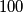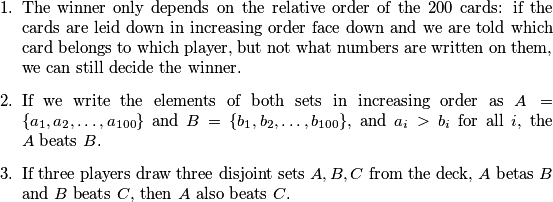IMO Shortlist 2014 problem C6

Kvaliteta:
Avg: 0,0
Težina:
Avg: 8,0

We are given an infinite deck of cards, each with a real number on it. For every real number$x$, there is exacly one card in the deck that has$x$ written on it. Now two players draw disjoint sets$A$ and$B$ of$100$ cards each from this deck. We would like to define a rule that declares one of them a winner. This rule should satisfy the following conditions:How many ways are there define such a rule? Here, we consider the rules as different if there exist two sets$A$ and$B$ such that$A$ beats$B$ according to the rule, but$B$ beats$A$ according to the other.

(Russia)

Izvor: https://www.imo-official.org/problems/IMO2014SL.pdf